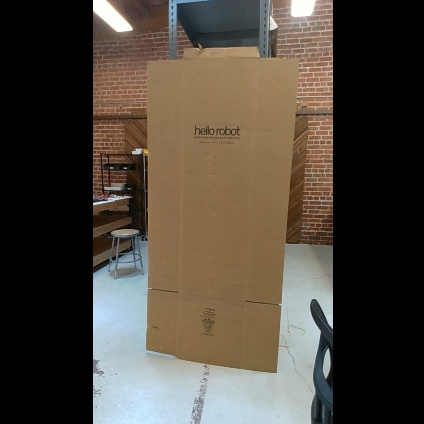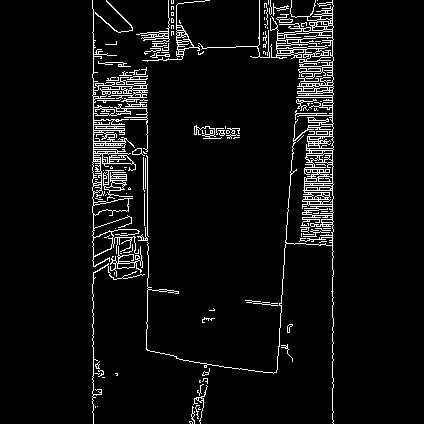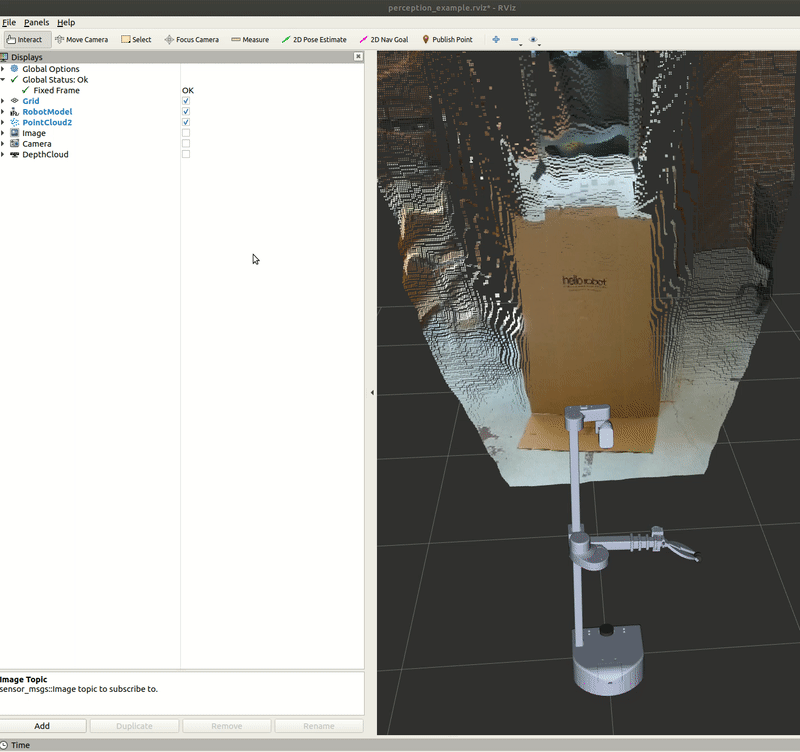# Example 7

In this example, we will review the image_view ROS package and a Python script that captures an image from the RealSense camera.Begin by running the stretch `driver.launch` file.

``````roslaunch stretch_core stretch_driver.launch
``````

To activate the RealSense camera and publish topics to be visualized, run the following launch file in a new terminal.

``````roslaunch stretch_core d435i_low_resolution.launch
``````

Within this tutorial package, there is an RViz config file with the topics for perception already in the Display tree. You can visualize these topics and the robot model by running the command below in a new terminal.

``````rosrun rviz rviz -d /home/hello-robot/catkin_ws/src/stretch_tutorials/rviz/perception_example.rviz
``````

## Capture Image with image_view

There are a couple of methods to save an image using the image_view package.

OPTION 1: Use the `image_view` node to open a simple image viewer for ROS sensor_msgs/image topics. In a new terminal, execute:

``````rosrun image_view image_view image:=/camera/color/image_raw_upright_view
``````

Then you can save the current image by right-clicking on the display window. By default, images will be saved as frame000.jpg, frame000.jpg, etc. Note, that the image will be saved to the terminal's current work directory.

OPTION 2: Use the `image_saver` node to save an image to the terminal's current work directory. In a new terminal, execute:

``````rosrun image_view image_saver image:=/camera/color/image_raw_upright_view
``````

## Capture Image with Python Script

In this section, we will use a Python node to capture an image from the RealSense camera. Execute the capture_image.py node to save a .jpeg image of the image topic `/camera/color/image_raw_upright_view`. In a terminal, execute:

``````cd ~/catkin_ws/src/stretch_tutorials/src
python3 capture_image.py
``````
An image named camera_image.jpeg is saved in the stored_data folder in this package.

### The Code

``````#!/usr/bin/env python3

import rospy
import sys
import os
import cv2

from sensor_msgs.msg import Image
from cv_bridge import CvBridge, CvBridgeError

class CaptureImage:
"""
A class that converts a subscribed ROS image to a OpenCV image and saves
the captured image to a predefined directory.
"""
def __init__(self):
"""
A function that initializes a CvBridge class, subscriber, and save path.
:param self: The self reference.
"""
self.bridge = CvBridge()
self.sub = rospy.Subscriber('/camera/color/image_raw', Image, self.callback, queue_size=1)
self.save_path = '/home/hello-robot/catkin_ws/src/stretch_tutorials/stored_data'

def callback(self, msg):
"""
A callback function that converts the ROS image to a CV2 image and stores the
image.
:param self: The self reference.
:param msg: The ROS image message type.
"""
try:
image = self.bridge.imgmsg_to_cv2(msg, 'bgr8')
except CvBridgeError as e:
rospy.logwarn('CV Bridge error: {0}'.format(e))

file_name = 'camera_image.jpeg'
completeName = os.path.join(self.save_path, file_name)
cv2.imwrite(completeName, image)
rospy.signal_shutdown("done")
sys.exit(0)

if __name__ == '__main__':
rospy.init_node('capture_image', argv=sys.argv)
CaptureImage()
rospy.spin()
``````

### The Code Explained

Now let's break the code down.

``````#!/usr/bin/env python3
``````
Every Python ROS Node will have this declaration at the top. The first line makes sure your script is executed as a Python3 script.

``````import rospy
import sys
import os
import cv2
``````

You need to import `rospy` if you are writing a ROS Node. There are functions from `sys`, `os`, and `cv2` that are required within this code. `cv2` is a library of Python functions that implements computer vision algorithms. Further information about cv2 can be found here: OpenCV Python.

``````from sensor_msgs.msg import Image
from cv_bridge import CvBridge, CvBridgeError
``````

The `sensor_msgs.msg` is imported so that we can subscribe to ROS `Image` messages. Import CvBridge to convert between ROS `Image` messages and OpenCV images.

``````def __init__(self):
"""
A function that initializes a CvBridge class, subscriber, and save path.
:param self: The self reference.
"""
self.bridge = CvBridge()
self.sub = rospy.Subscriber('/camera/color/image_raw', Image, self.callback, queue_size=1)
self.save_path = '/home/hello-robot/catkin_ws/src/stretch_tutorials/stored_data'
``````

Initialize the CvBridge class, the subscriber, and the directory where the captured image will be stored.

``````def callback(self, msg):
"""
A callback function that converts the ROS image to a cv2 image and stores the
image.
:param self: The self reference.
:param msg: The ROS image message type.
"""
try:
image = self.bridge.imgmsg_to_cv2(msg, 'bgr8')
except CvBridgeError as e:
rospy.logwarn('CV Bridge error: {0}'.format(e))
``````

Try to convert the ROS Image message to a cv2 Image message using the `imgmsg_to_cv2()` function.

``````file_name = 'camera_image.jpeg'
completeName = os.path.join(self.save_path, file_name)
cv2.imwrite(completeName, image)
``````

Join the directory and file name using the `path.join()` function. Then use the `imwrite()` function to save the image.

``````rospy.signal_shutdown("done")
sys.exit(0)
``````

The first line of code initiates a clean shutdown of ROS. The second line of code exits the Python interpreter.

``````rospy.init_node('capture_image', argv=sys.argv)
CaptureImage()
``````

The next line, `rospy.init_node(NAME, ...)`, is very important as it tells rospy the name of your node -- until rospy has this information, it cannot start communicating with the ROS Master.

Note

The name must be a base name, i.e. it cannot contain any slashes "/".

Instantiate the `CaptureImage()` class.

``````rospy.spin()
``````

Give control to ROS. This will allow the callback to be called whenever new messages come in. If we don't put this line in, then the node will not work, and ROS will not process any messages.

## Edge Detection

In this section, we highlight a node that utilizes the Canny Edge filter algorithm to detect the edges from an image and convert it back as a ROS image to be visualized in RViz. In a terminal, execute:

``````cd ~/catkin_ws/src/stretch_tutorials/src
python3 edge_detection.py
``````

The node will publish a new Image topic named `/image_edge_detection`. This can be visualized in RViz and a gif is provided below for reference.### The Code

``````#!/usr/bin/env python3

import rospy
import sys
import os
import cv2

from sensor_msgs.msg import Image
from cv_bridge import CvBridge, CvBridgeError

class EdgeDetection:
"""
A class that converts a subscribed ROS image to a OpenCV image and saves
the captured image to a predefined directory.
"""
def __init__(self):
"""
A function that initializes a CvBridge class, subscriber, and other
parameter values.
:param self: The self reference.
"""
self.bridge = CvBridge()
self.sub = rospy.Subscriber('/camera/color/image_raw', Image, self.callback, queue_size=1)
self.pub = rospy.Publisher('/image_edge_detection', Image, queue_size=1)
self.save_path = '/home/hello-robot/catkin_ws/src/stretch_tutorials/stored_data'
self.lower_thres = 100
self.upper_thres = 200
rospy.loginfo("Publishing the CV2 Image. Use RViz to visualize.")

def callback(self, msg):
"""
A callback function that converts the ROS image to a CV2 image and goes
through the Canny Edge filter in OpenCV for edge detection. Then publishes
that filtered image to be visualized in RViz.
:param self: The self reference.
:param msg: The ROS image message type.
"""
try:
image = self.bridge.imgmsg_to_cv2(msg, 'bgr8')
except CvBridgeError as e:
rospy.logwarn('CV Bridge error: {0}'.format(e))

image = cv2.Canny(image, self.lower_thres, self.upper_thres)
image_msg = self.bridge.cv2_to_imgmsg(image, 'passthrough')
self.pub.publish(image_msg)

if __name__ == '__main__':
rospy.init_node('edge_detection', argv=sys.argv)
EdgeDetection()
rospy.spin()
``````

### The Code Explained

Since there are similarities in the capture image node, we will only break down the different components of the edge detection node.

Define the lower and upper bounds of the Hysteresis Thresholds.

``````image = cv2.Canny(image, self.lower_thres, self.upper_thres)
``````

Run the Canny Edge function to detect edges from the cv2 image.

``````image_msg = self.bridge.cv2_to_imgmsg(image, 'passthrough')
``````

Convert the cv2 image back to a ROS image so it can be published.

``````image_msg.header = msg.header
self.pub.publish(image_msg)
``````

Publish the ROS image with the same header as the subscribed ROS message.